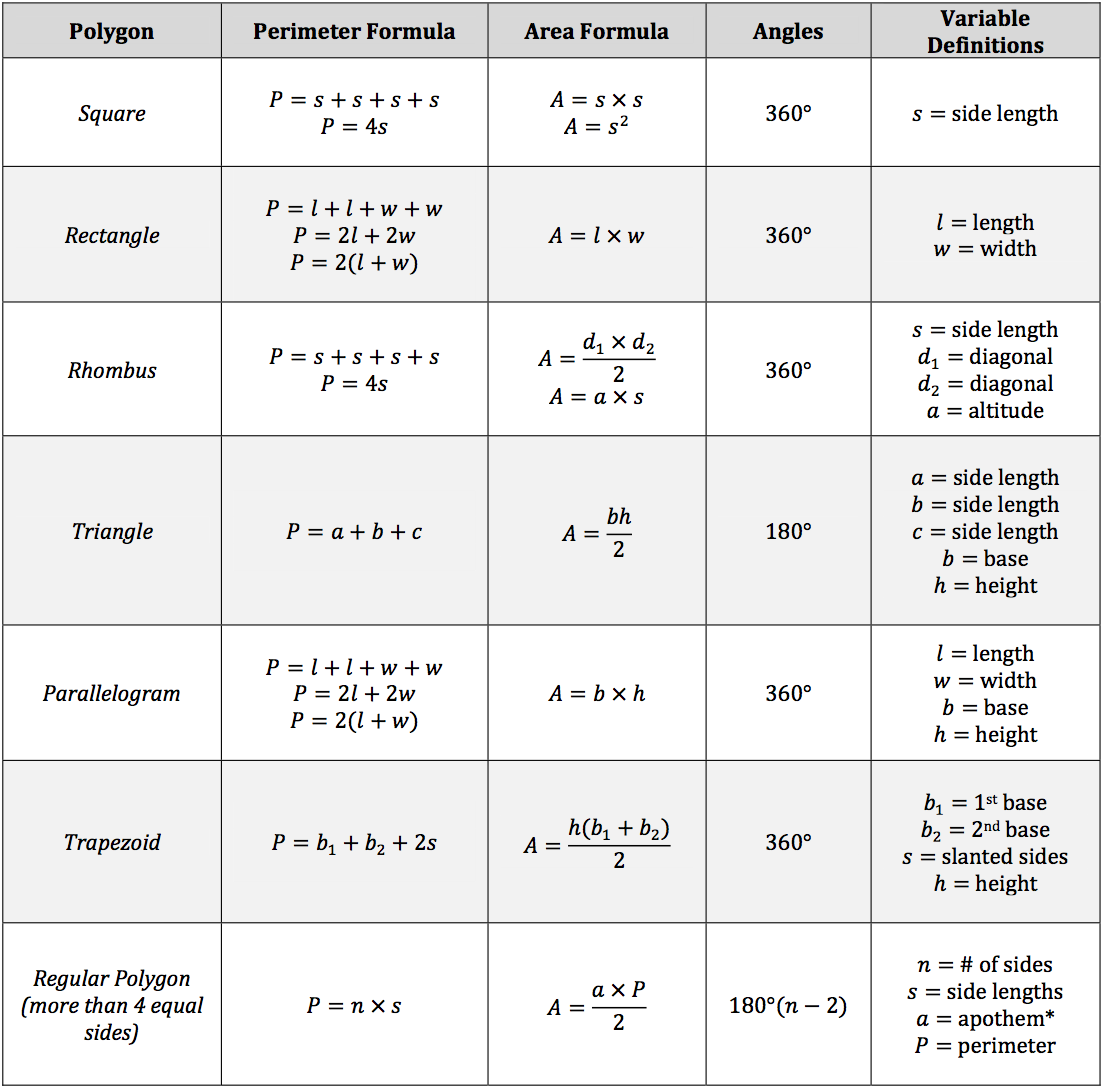Polygons are two‐dimensional shapes that are formed by three or more straight sides. You will be analyzing these geometric figures to find the total degree value of a polygon, the perimeter of a polygon, and the area of a polygon.

• The perimeter is the measurement of the sum of the side lengths of a polygon.
• The area is the measurement of the inner portion of the polygon.*Apothem is perpendicularly drawn from the center of the polygon to the midpoint of a side.